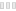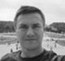# Modern Calculator

CleanA review by AndrewArithmetic calculations are made easier by using either a manual or digital calculators. The application “Modern Calculator” is a tool that is used to perform scientific and mathematical calculations  with a graphical presentation. It has all the formulas needed to make complex calculations. You only need to highlight the function and the program automatically carrys out the mathematical functions.  It performs all mathematical calculations on various topics. They include Trigonometry, Calculus, Differentiation, Integration, Vectors and Algebra among others. All you need to do is to enter the variables and the calculator does the rest of the calculations. However, you need to be keen to key in the right variables. For example, in drawing graphs you should not confuse the X and the Y variables, otherwise the graph will not be the correct.

All the calculations are saved and used for future reference. It solves equations of one or two unknowns. It also converts Roman numerical to arithmetic quantities. It can also solve algebraic expressions. Additionally, it can convert unit measurements to different scalar quantities in the same genre. Finally, it can design a 2D graphical presentation that can be used for interpretation. As long as you know the mathematical basics you can comfortably use the program “Modern Calculator”.

It is part from calculators category and is licensed as shareware for Windows 32-bit and 64-bit platform and can be used as a free trial until the trial period will end. The Modern Calculator 2.0 demo is available to all software users as a free download with potential restrictions compared with the full version.

 Share Give a rating(1 votes, average: 5.00 out of 5)login to voteLoading... Author Amazing Software Solutions Last Updated On April 18, 2017 Version 2.0 Runs on Windows 10 / Windows 8 / Windows 7 / Windows Vista / XP Total downloads 1,545 License Free to try (Shareware) File size 1,38 MB Filename moderncalc.exe

### Similar programsjExacter
A high precision calculator
SoftFamous
X